# Chapter XI: Privacy in Multidimensional Databases

Francesco Malvestuto, University of Rome–La Sapienza

Italy

Marina Moscarini, University of Rome–La Sapienza

Italy

When answering queries that ask for summary statistics, the query-system of a multidimensional database should guard confidential data, that is, it should avoid revealing (directly or indirectly) individual data, which could be exactly calculated or accurately estimated from the values of answered queries. In order to prevent the disclosure of confidential data, the query-system should be provided with an auditing procedure which, each time a new query is processed, checks that its answer does not allow a (knowledgeable) user to disclose any sensitive data. A promising approach consists in keeping track of (or auditing) answered queries by means a dynamic graphical data structure, here called the answer map, whose size increases with the number of answered queries and with the number of dimensions of the database, so that the problem of the existence of an efficient auditing procedure naturally arises. This chapter reviews recent results on this problem for "additive" queries (such as COUNT and SUM queries) by listing some polynomially solvable problems as well as some hard problems, and suggests directions for future work.

## INTRODUCTION

The explosive increase in access to "statistical databases" has aroused concerns on the compromise of individual privacy. A statistical database (Denning, 1982; Date, 1983; Ullman, 1983; Michalewicz, 1991) is a database which contains information about individuals (companies, organizations, etc.) and whose users are only allowed to access summary statistics, but "sensitive" ones, that is, summary statistics that could lead to the exact or approximate disclosure of confidential data of single individuals. A naïve policy for guarding the confidentiality of individual data consists in leaving unanswered queries asking for sensitive summary statistics. This policy is not satisfactory because, given a set of non-sensitive summary statistics, there is a more-or-less large set of summary statistics that are implicitly released in that they can be computed in an exact or approximate way so that, if one of such summary statistics were sensitive, then the confidentiality of some individual data would be compromised. To guarantee privacy of individual records, a mechanism of inference control must be embodied in the statistical database interface according to some security policy, which can be said to be effective only if sensitive summary statistics are neither explicitly nor implicitly released.

We can imagine how an ideal control method should work. If Q is the set of previously answered queries and Q is a new query, then the value of Q is initially "locked." Next, Q is tested for sensitivity; if the test is positive, the answer to Q will be denied; otherwise, the sensitivity test is applied to each of the summary statistics that would be implicitly revealed if Q were answered; if none of these summary statistics comes out to be sensitive, then and only then the value of Q is "unlocked" and Q is answered. Such a security procedure raises the computational problem of finding out the summary statistics that are implicitly revealed from the values of a given set of answered queries. This problem, sometimes called the "inference problem," proves to be hard in the general case and has been solved in an efficient way only under certain restrictive assumptions. The complexity of the inference problem essentially depends on the query type and on the sensitivity criterion in use. The query type is defined by the following three parameters: the aggregation function (count, sum, average, max, min, etc.), the data type (real, nonnegative real, integer, nonnegative integer, etc.) and the data structure (simple if the answer to the query consists of a scalar, and complex if the answer consists of a structured data such as a table). Note that a complex query can be always thought of as a set of simple queries, and that average queries can be thought of being couples of queries. As to the sensitivity criteria, several proposals exist (Adam & Wortmann, 1989; Adam, Gangopadyay & Holowczak, 1999).

In this chapter, we review some recent results on the computational complexity of the inference problem for simple count and sum queries, which will be referred to as simple "additive queries," with data of real and nonnegative real type.

The inference problem for additive queries with data of integer and nonnegative integer type is far more complicated, and some results can be found in Chin (1986) and Kleinberg, Papadimitriou, & Raghavan (2000), where max and min queries are also discussed. It is worth mentioning that a similar inference problem arises in the publication of statistical two- and three-dimensional tables; it is then called the problem of the "statistical disclosure control" (Cox, 1980, 1981; Kelly, Golden & Assad, 1992; Carvalho, Dellaert, & Osorio, 1994; Irvin & Jerrum, 1994), and surveys can be found in Schackis (1993), Cox & Zayatz (1995), Willenborg & de Waal (1996, 2000), and Roehrig (2001). Although most results of the theory of statistical disclosure control apply to complex additive queries on a statistical database, there are two main aspects of the inference problem in statistical databases that make it harder: one is the on-line characteristic of statistical query processing, and the other is that the statistical disclosure control of a table aims at protecting sensitive cells only, and not general forms of sensitive information which might consist of sensitive sets of cells.

To give a taste of the security issues connected with statistical data, we now present some examples of statistical disclosure control on tables which serve to introduce basic notions. Let us suppose that the economic division of a census bureau has collected a wide range of data and wishes to publish these data without violating confidentiality laws. Normally, economic data is published by geography and standard industrial classification (SIC) codes. For example, Table 1 shows state-level data for various types of food stores.Table 1

SIC

Number of Establishments

Value of Sales (\$)

54

All Food Stores

347

200,900

541

Grocery

333

196,000

542

Meat and Fish

11

1,500

543

Fruit Stores

2

2,400

544

Candy

1

1,000This table shows that only one establishment reported candy store sales for this state. If this table were published, any data user would know the candy establishment's precise sales value. Also, this table shows only two establishments reporting fruit store sales. Either of these two establishments, knowing their own sales figure, could calculate the other establishment's precise sales value. Thus, publishing this table would result in a violation of the privacy right. Cell values such as these are considered sensitive, and must not be published. A way to prevent the disclosure of sensitive values is to simply not publish the values. When this table is published, the sensitive cell values are suppressed or "obscured" (primary suppression). Table 2 shows an incomplete table where the two sensitive cell values have been suppressed.Table 2

SIC

Number of Establishments

Value of Sales (\$)

54

All Food Stores

347

200,900

541

Grocery

333

196,000

542

Meat and Fish

11

1,500

543

Fruit Stores

2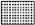544

Candy

1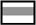Generally speaking, if only sensitive cells of a table are suppressed, users could derive their contents from non-sensitive data owing to the data additivity. Therefore, to fully protect the contents of suppressed sensitive cells, additional cells must be suppressed (complementary suppression). Of course, one must be certain that no suppressed cell values can be derived exactly. It is rarely sufficient to merely look at a table and determine that the complementary suppression scheme fully protects all sensitive cell values. Often a two-dimensional table seems to have an adequate number of complementary suppressions, but mathematical manipulations may reveal a suppressed cell value. For example, consider Table 3 and assume that cells (2, 1), (3, 2), (3, 3), and (4, 3) are sensitive.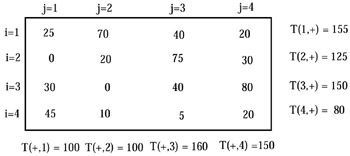Table 4 is obtained from Table 3 by suppressing the four sensitive cells (primary suppressions), and cells (1, 2), (1, 4), (2, 3), (3, 4), and (4, 1) (complementary suppressions).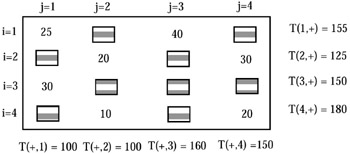At first, it could seem that there is a sufficient number of suppressions to protect the values of all sensitive cells. However, the value T(3, 3) of the sensitive cell (3, 3) can be easily determined as follows: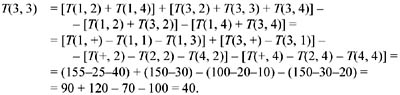Moreover, even when no sensitive cell value can be determined, one can always estimate every suppressed value if the data is of nonnegative type. For example, consider Table 5 where all interior cells are obscured.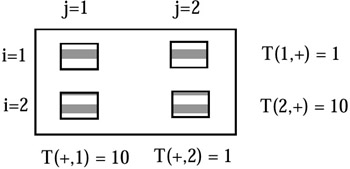If the data is of real type, then the feasible values of each suppressed cell range from – to +. But, if the data is of nonnegative real type, then with some linear programming, a data user can find out that the feasible values for each suppressed cell range in a bounded interval; for example, the feasible values for the cell value T(1, 1), T(1, 2), and T(2, 2) span the interval [0, 1] and the feasible values for the cell value T(2, 1) span the interval [9, 10]. Worst of all, if the data is of nonnegative integer type, there are exactly two feasible values for each cell.

Table 1 showed an obvious type of sensitive cell: only one or two firms contributed at a cell. A not-so-obvious sensitivity occurs when more than two firms contribute to a cell, but one firm is able to estimate the data for another firm very closely. With magnitude data, if the data is of nonnegative type, a stronger sensitivity criterion is commonly used by census bureaus, namely, the (n, k%) dominance rule, where n is a positive integer and k is a percentage. According to the (n, k%) dominance rule, a cell (i, j) is considered sensitive if the sum of n or fewer individual contributions to the cell value T(i, j) accounts for more than k% of T(i, j). If this is the case, a coalition of at most n–1 individuals in cell (i, j) can get an accurate estimate of the largest contribution to T(i, j).

For frequency tables, that is, with count data, the n threshold rule is commonly used; accordingly, a cell (i, j) is considered sensitive if T(i, j) n.

To sum up, a security policy is defined by two parameters which are kept secret to the data users: the sensitivity criterion (e.g., the dominance criterion, the threshold criterion, etc.) and the protection type (from exact or approximate inference).

In the next sections, we discuss the computational issues connected with the implementation of a security policy in an on-line database environment. The chapter is organized as follows. First we introduce some basic definitions on additive queries. The next section contains the formal framework for the inference problem and the notion of an "answer map," which is the tool of a database user to disclose sensitive information. We then deal with "graphical" answer maps, and efficient algorithms are given. A few notions of safety are introduced, and the computational scheme of an auditing procedure is presented. The next section deals with two-dimensional tables, and the final sections contain future direction and conclusions.Multidimensional Databases: Problems and Solutions
ISBN: 1591400538
EAN: 2147483647
Year: 2003
Pages: 150